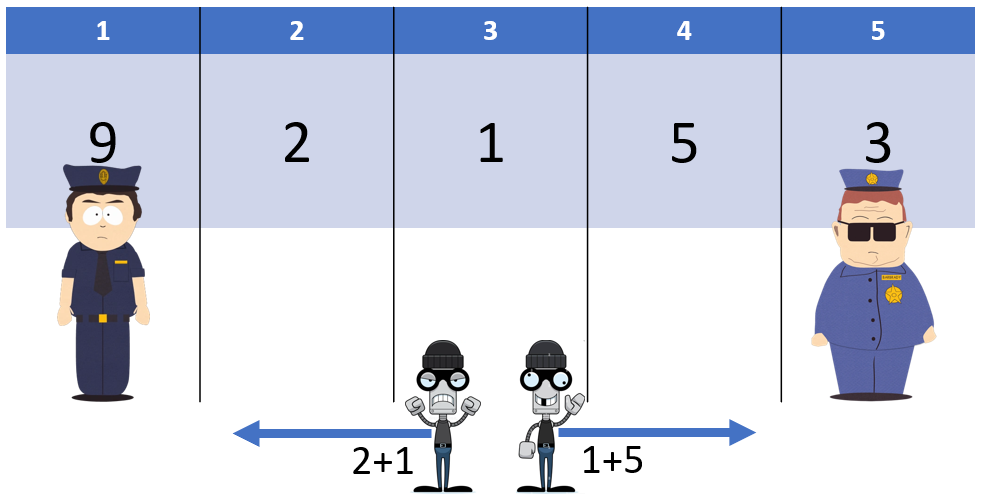# Bank Robberyrana_hossainDIU Take-Off Programming...57/66/131
Limits 1s, 512 MB

A robber attempted to rob TS(Taka Sesh) Bank but failed to complete the task. However, he managed to open all the vaults successfully.

Bob is a scientist in a tech giant company specializing in developing AI-based robots. His work has earned him considerable fame in his field. Interestingly, Bob is also a client of TS Bank (Taka Sesh Bank), and like many others, he is also greedy for money. Taking advantage of the failed robbery, Bob decides to steal money from the vaults, sending an AI-based robot named ‘Alpha’ to TS bank to steal that money.

There are $n$ vaults arranged in a line left to right, where the $i^{th} \: (1 \le i \le n)$ vault has $x_i$ money in it.

There are two security guards, one of them guards the $b^{th}$ vault, and the other one guards the $c^{th}$ vault. The two guards have strict instructions not to leave their guarding posts.

Alpha can go only left or right. Alpha won’t visit a vault more than once. Whenever Alpha is in a vault, he will steal all the money in that vault.

Alpha starts stealing from the $a^{th}$ vault. Alpha is at a disadvantage that the $a^{th}$ vault is one of the vaults which are between the $b^{th}$ and the $c^{th}$ vault. Meaning Alpha has a guard in one of the vaults left of him and the other one guarding one of the vaults right of him, meaning $b.

Note that Alpha cannot steal from a guarded vault. So, Alpha stops stealing and escapes the bank if it encounters a guard.

Now you have to print what is the maximum amount of money Alpha can steal from the TS Bank.

## Input

The first line of input will contain four integers $n, a, b, c \: (3\le n \le 10^5; \: 1 \le b < a < c \le n)$, separated by spaces — denoting the number of vaults and the positions of Alpha, the first security guard, and the second security guard, respectively.

The next line of input contains $n$ space separated integers $x_1, x_2,..., x_n \: (0 \le x_i \le 10^3)$, denoting that the $i^{th}$ vault has $x_i$ amount of money.

## Output

Output a single integer — denoting the maximum amount that Bob can steal from the bank.

Check out the samples for clarification.

## Sample

InputOutput
5 3 1 5
9 2 1 5 3

6


Explanation:If Alpha moves to the left, it can steal from the $2^{nd}$ and $3^{rd}$ vaults, resulting in a total of $3$.

On the other hand, if it moves to the right, it can steal from the $3^{rd}$ and $4^{th}$ vaults, resulting in a total of $6$. Here, Alpha cannot steal from the $2^{nd}$ vault because he has already visited $3^{rd}$ vault, to steal from the $2^{nd}$ vault he has to go through $3^{rd}$ which he can’t. So, final value is $6$.

### Submit